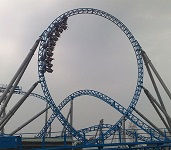Skip to main content
$$\require{cancel}$$

# 03: Two-Dimensional KinematicsPrince George's Community College General Physics 1030 PHYS 1030 Prof. Neeharika Thakur 7: Momentum & Collisions    8: Static Equilibrium Elasticity & Torque      9: Rotational Kinematics     10: Fluids     11: Fluid Dynamics

• 3.1: Motion in Two Dimensions
An object moving with constant velocity must have a constant speed in a constant direction.
• 3.2: Vectors
Vectors are geometric representations of magnitude and direction and can be expressed as arrows in two or three dimensions.
• 3.3: Projectile Motion
Projectile motion is a form of motion where an object moves in parabolic path; the path that the object follows is called its trajectory.
• 3.4: Multiple Velocities
Relative velocities can be found by adding the velocity of the observed object to the velocity of the frame of reference it was measured in.

• Was this article helpful?